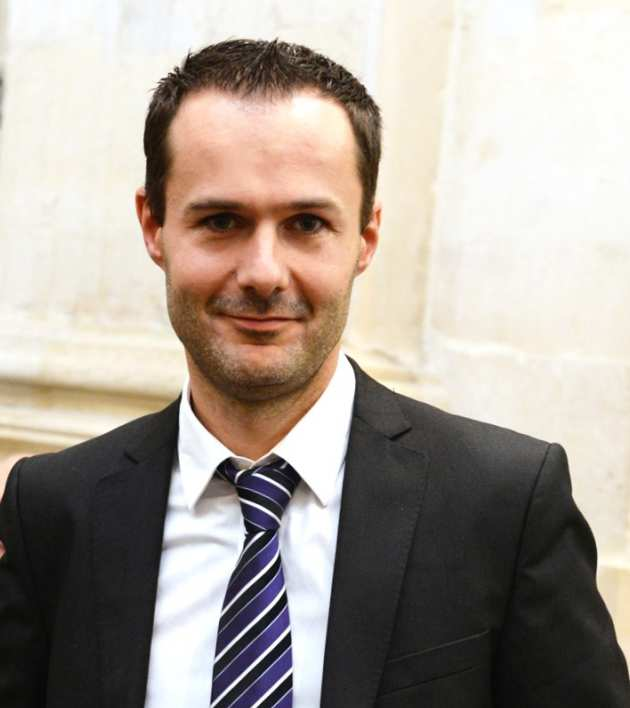The Department is delighted to welcome Francis Filbet (Univeristy of Toulouse) as a Nelder Fellow in January 2016.Background: Francis Filbet's research activity focuses mainly on numerical simulations and analysis of phenomena
described by time dependent nonlinear partial differential equations, in particular by
parabolic, hyperbolic and kinetic models. These equations express fundamental laws of physics and are of great importance in many modern applications that range from gas dynamics, plasma and quantum physics to traffic flows, economy and biology, to mention only a few relevant examples.

View Prof Fibert's webpage

Abstract: Francis Filbet will deliver a mini-course on analytical and numerical methods for kinetic equations and transport phenomena. In this course the design and the mathematical analysis of numerical methods for kinetic equations will be considered. Kinetic theory represents a way of describing the time evolution of a system consisting of a large number of particles. This class of models is an essential tool to make the rigorous link between a microscopic description and a macroscopic description of the physical reality. Furthermore, due to the high number of dimensions and their intrinsic physical properties, the construction of numerical methods represents a challenge and requires a careful balance between accuracy and computational complexity.

We will focus on a classical system dealing with Coulombian (or Newtonian) interactions between particles in the presence of a strong external magnetic field. We first present the analysis of the Vlasov-Poisson system (weak and strong solutions) and give the rigorous asymptotic analysis to get reduced transport models, that is, the guiding center system.

Then we review the basic numerical techniques based on particle methods for dealing with such equations. In addition we give an overview of the current state of the art of numerical methods for kinetic equations.

Lecture 1: Monday 11 January, 16:00-18:00, Huxley 130

An introduction to kinetic theory

-       The problem of particle dynamics.

-       Some fundamental models of interacting particles: Vlasov-Poisson, Vlasov-Maxwell systems

-       Existence of weak solution for the Vlasov-Poisson system

Lecture 2: Wednesday 13 January, 16:00-18:00, Huxley 130

The Vlasov-Poisson system with a strong external magnetic field

-       Formal asymptotic analysis and guiding center approximation

-       Rigorous analysis by F. Golse and L. Saint-Raymond

Lecture 3: Wednesday 20 January, 16:00-18:00, Huxley 130

Particle methods for Vlasov equation

-       Particle-in-Cell methods

-       Analysis of particle methods in the presence of a strong external magnetic field

-       Numerical simulations

Follow the link to find out further information on Prof Filbet's website